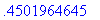Error: (in int) wrong number (or type) of parameters in function iquo

This error is caused by a bug in Release 4 affecting some integrals with nested radicals.

> int(sqrt(x+sqrt(x)),x);

Error, (in int) wrong number (or type) of parameters in function iquo

> int(sqrt(1+y^(-2/3)),y=1..2);

Error, (in int) wrong number (or type) of parameters in function iquo

The bug is fixed in Release 5.

A work-around is to apply a change of variables, using changevar from the student package.

> with(student,changevar);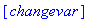> changevar(sqrt(x)=t,Int(sqrt(x+sqrt(x)),x),t);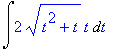> value(");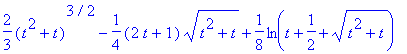> F:=subs(t=sqrt(x),");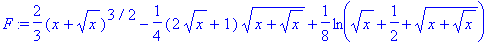It's prudent to check an integral by differentiating it and comparing to the original integrand.

> diff(F,x);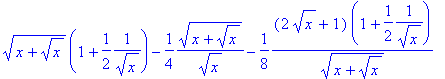> normal("-sqrt(x+sqrt(x)));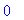In the second example:

> changevar(y^(-2/3)=t,Int(sqrt(1+y^(-2/3)),y=1..2),t);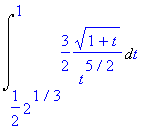> value(");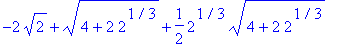A definite integral can be checked by comparing it to a numerical approximation.

> evalf(");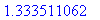> evalf(Int(sqrt(1+y^(-2/3)),y=1..2));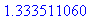Confirming the necessity of checking the result, another bug produces incorrect results for very similar integrals:

> J:=changevar(y^(-2/3)=t,Int(sqrt(1-y^(-2/3)),y=1..2),t);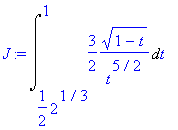> value(J);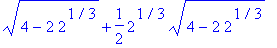> evalf(");The numerical approximation is

> evalf(Int(sqrt(1-y^(-2/3)),y=1..2));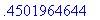In this case the correct result would be produced by combining the fractional powers.

> Int(3/2*sqrt((1-t)/t^5),t = 1/2*2^(1/3) .. 1);> value(");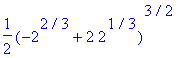> evalf(");